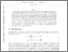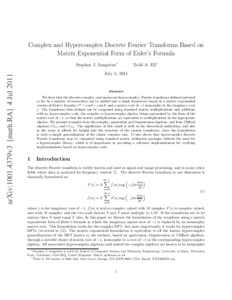# Complex and hypercomplex discrete Fourier transforms based on matrix exponential form of Euler's formula

Sangwine, SJ and Ell, TA (2012) 'Complex and hypercomplex discrete Fourier transforms based on matrix exponential form of Euler's formula.' Applied Mathematics and Computation, 219 (2). 644 - 655. ISSN 0096-3003Preview
Text
1001.4379v3.pdf - Accepted Version

Download (232kB) | Preview

## Abstract

We show that the discrete complex, and numerous hypercomplex, Fourier transforms defined and used so far by a number of researchers can be unified into a single framework based on a matrix exponential version of Euler's formula ejθ=cosθ+jsinθ, and a matrix root of -1 isomorphic to the imaginary root j. The transforms thus defined can be computed using standard matrix multiplications and additions with no hypercomplex code, the complex or hypercomplex algebra being represented by the form of the matrix root of -1, so that the matrix multiplications are equivalent to multiplications in the appropriate algebra. We present examples from the complex, quaternion and biquaternion algebras, and from Clifford algebras Cℓ1 ,1 and Cℓ2 ,0. The significance of this result is both in the theoretical unification, which permits comparisons between transforms in different hypercomplex algebras, and also in the scope it affords for insight into the structure of the various transforms, since the formulation is such a simple generalization of the classic complex case. It also shows that hypercomplex discrete Fourier transforms may be computed using standard matrix arithmetic packages without the need for a hypercomplex library, which is of importance in providing a reference implementation for verifying faster implementations based on hypercomplex code. © 2012 Elsevier Inc. All rights reserved.

Item Type: Article Q Science > QA MathematicsQ Science > QA Mathematics > QA75 Electronic computers. Computer science Faculty of Science and Health > Computer Science and Electronic Engineering, School of Jim Jamieson 15 Feb 2013 11:09 13 Mar 2019 11:15 http://repository.essex.ac.uk/id/eprint/5558

### Actions (login required)View Item